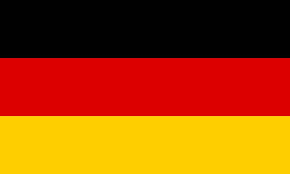# Free online math calculators

 Search through Mathepower for the right calculator.## Welcome to Mathepower!

Mathepower is a german website, established 2002. It is specialized on online-calculators. Just enter your exercise and it will be solved. In 2019 we started to make this website available for visitors from foreign countries.

## Take a quick look?

Here are some of our calculators that we'd like to recommend:

## New: Geometry renewed!

Geometry calculators are now also solving equations step-by-step using formulas. Take a look for example at right-angled triangles or cylinders

## Analysis

You waited quite some time for it, but since 2017 Mathepower also helps you with analysis. For example, it can do curve sketching , is also able to find turning, saddle and inflection points. The equation solver can also, of course, find roots of arbitrary functions.
FAQ:

## What can Mathepower do?

Mathepower contains calculators for nearly every mathematical topic. You don't just get your exercise solved, but get also the steps explained. So you can
• simply enter your exercise and get it explained, e.g. here for equations